# 0.展示PTA总分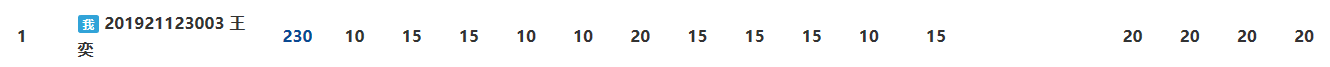# 1.本章学习总结

## 1.1 学习内容总结

• 指针做循环变量做法：
char *p;
for(p=a;p<a+n;p++)

• 字符指针如何表示字符串
char *str="abcdef";

• 动态内存分配
• 我们需要利用动态内存分配函数来分配所需要的存储空间，我们学过的动态内存分配函数有malloc（）和calloc（）
• 两个动态内存分配函数的区别是：malloc（）对所分配的存储块不做任何事，calloc（）对整个区域进行初始化
• 值得注意的是，这两个函数的类型都是void *，所以在将分配到的内存首地址赋给一个指针变量时，要强制类型转换，例如：
int *p;
p=(int *)malloc(n*sizeof(int));

• 动态申请的内存在使用完后要记得释放，动态存储释放函数为free（）
• 指针数组及其应用
• 如果数组中的各个元素都是指针类型，用于存放内存地址，那么这个数组就是指针数组，指针数组的数组名是一个二级指针，是指向指针的指针。
• 应用：用指针数组来存储字符串，数组里的内容只是每一个字符串的首地址，节省了数组所占的内存，而且对于字符串的引用也更加便捷。
• 二级指针、行指针
• 二级指针是指向指针的指针，表示方法如下：
int **p;

其中，p和p都表示地址，p指向p，而*p指向内容。**p则表示内容。
• 函数返回值为指针
• 只有函数本身的类型为指针类型，函数返回值才能为指针。
• 返回的指针最好不要指向函数内部定义的变量，因为函数内部定义的变量为局部变量，在函数运行结束后，这些变量会自动销毁。

## 1.2 本章学习体会

• 都说指针是C的灵魂，通过本章的学习，确实能感受到在一些问题的解决和参数的传递上，使用指针是非常便利的，但同时指针也确实不易理解，尤其是二级指针，这需要我们多做题，才能熟练地运用指针。
• 代码量约1000行左右。

# 2.PTA实验作业

## 2.1 合并两个有序数组

#### 2.1.1 伪代码

开辟一个数组c用于存放合并后的数组
i表示a数组的下标，j表示b数组的下标，k表示c数组的下标
for i=j=k=0 to a数组或b数组全部存入c数组中 do
if a[i]<b[j] then a[i]放入c数组，i++，k++；
else b[j]放入c数组，j++，k++；
end if
end for
while(i<m) a数组剩下元素放入c数组中
while(j<n) b数组剩下元素放入c数组中


#### 2.1.2 代码截图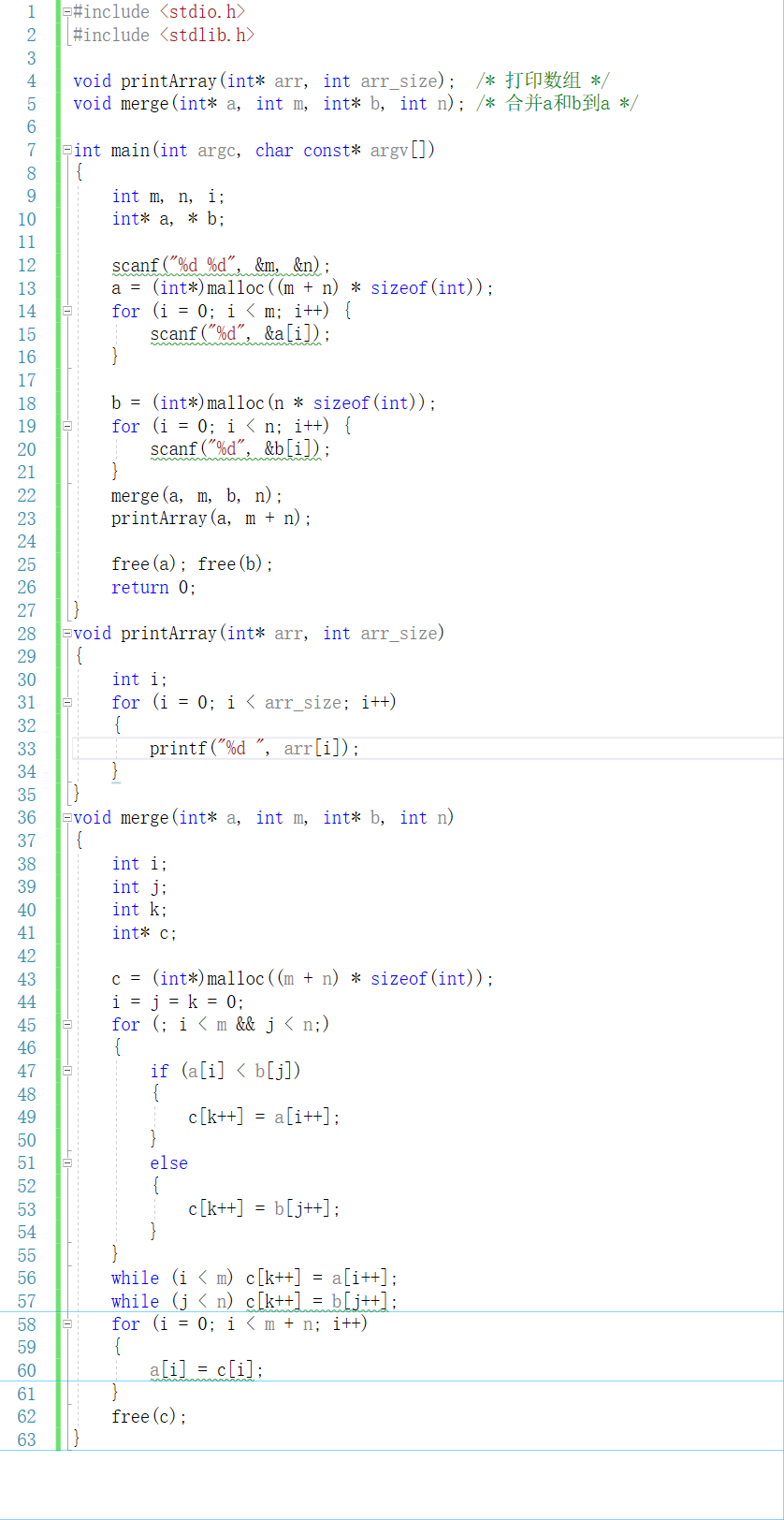#### 2.1.4 PTA提交列表及说明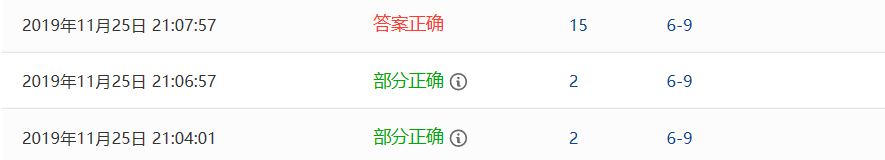1.部分正确：第一次我没有定义c数组去存放合并后的数组，而是在a数组中直接有序插入b数组的各个元素，这就导致了在数据较大时，我的程序会运行超时。
2.部分正确：这一次我只是在上一个程序的基础上做了一点点的修改，依然没有解决运行超时的问题。
3.答案正确：在听完老师的讲解后，我定义了一个c数组来存放合并后的数组，大大提高了程序运行的效率，最后答案正确。


## 2.2 删除字符串中的子串

#### 2.2.1 伪代码

利用strlen()函数计算出子串的长度len
len=strlen(substr)-1;

while locPtr!=NULL do
for p=locPtr to *p do
*p = *(p + len);
if (*p == 0 || *p == '\n') then break;
end if
end for
*p=0;


#### 2.2.2 代码截图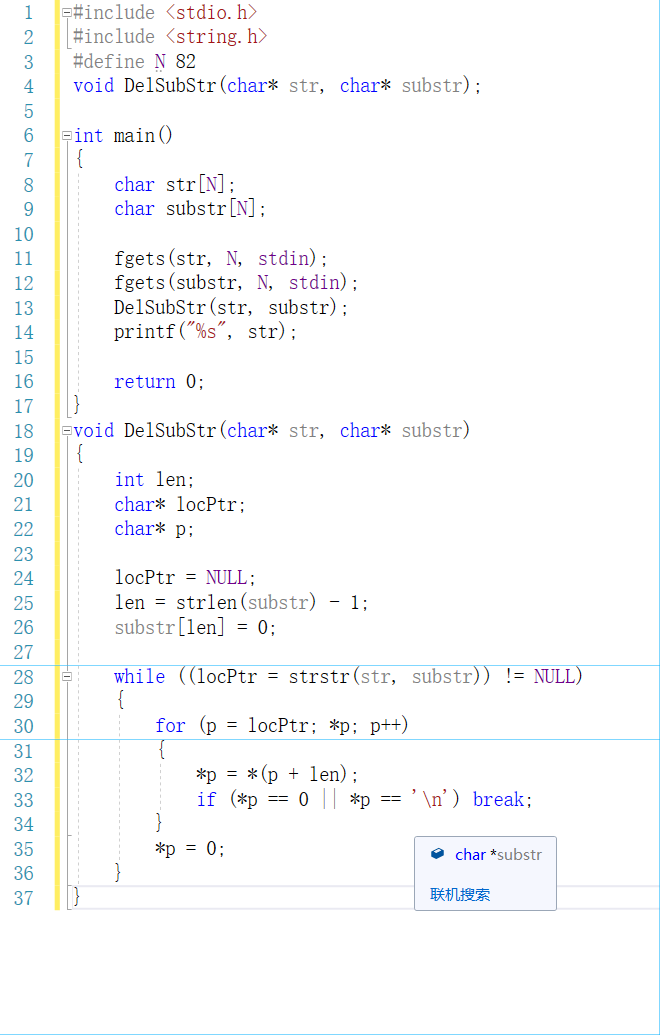#### 2.2.4 PTA提交列表及说明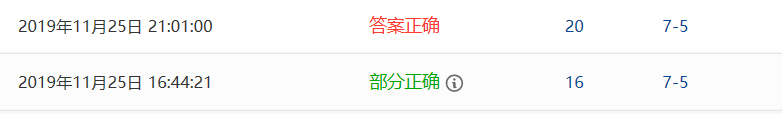1.部分正确：最初我不知道有strstr()这个函数，于是自己写了循环去查找子串，导致在程序运行时会出现一些错误，比如在需要全删空的情况下，我的程序就无法做到。
2.答案正确：在利用了strstr()函数后，答案正确。


## 2.3 说反话

#### 2.3.1 伪代码

从最后一位开始倒着遍历数组

if count>0 then 输出一个空格
end if
for i=head to i=tail do 输出a[i]
end for


#### 2.3.2 代码截图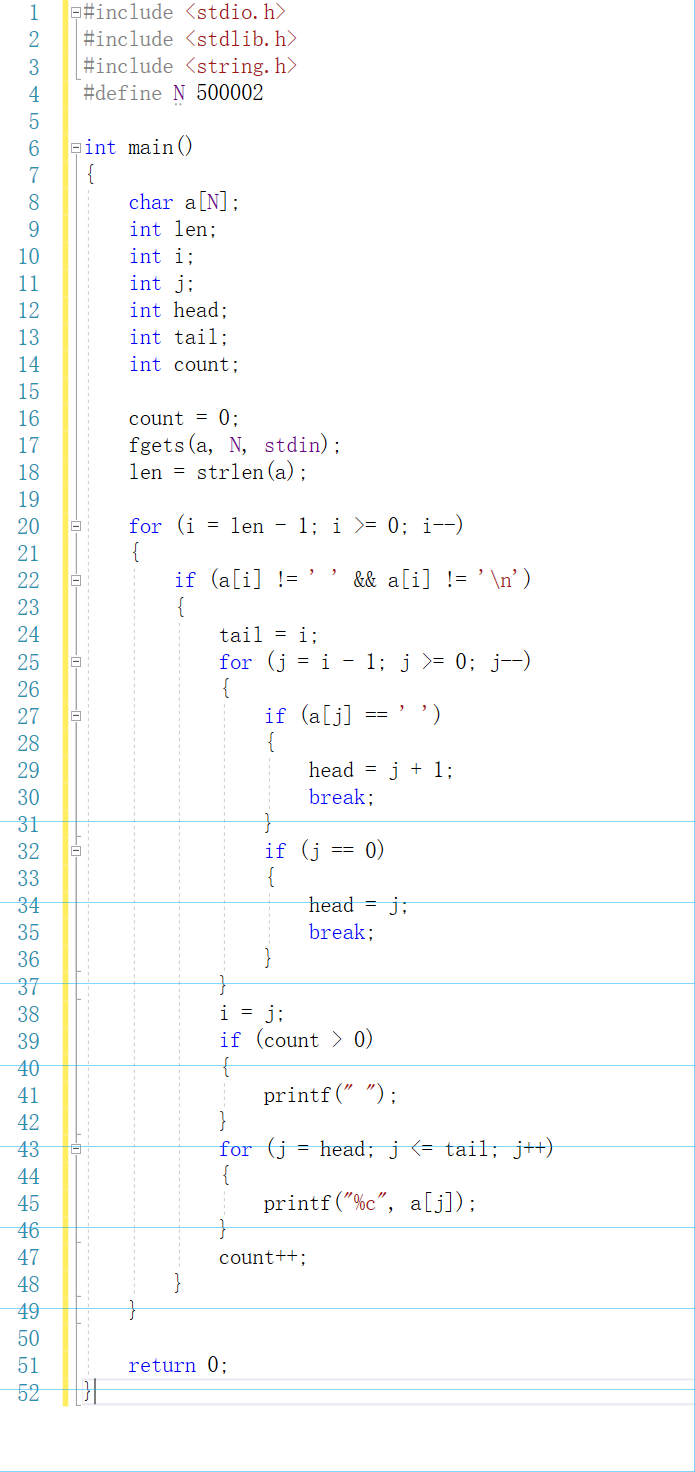#### 2.3.4 PTA提交列表及说明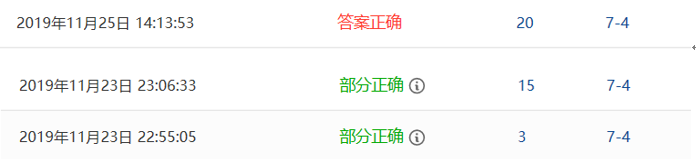2.部分正确：我的N值为500000，当句子长度为最大时，会有个别字符无法收入，导致答案错误。
3.答案正确：把宏定义改为“#define N 500002”，答案正确。


# 3.阅读代码

/*题目为：罗马数字转整数*/
#define nI 1  //直接用define I 1，好像会有歧义
#define nV 5
#define nX 10
#define nL 50
#define nC 100
#define nD 500
#define nM 1000

int romanToInt(char* s)
{
int num = 0, flag = 0;
while(*s != NULL)
{
if(*s == 'I' && (*(s + 1) == 'V' || *(s + 1) == 'X'))     //接下来的这三个if都有特殊含义，所以flag=1，普通情况属于flag=0
{
flag = 1;
switch(*(s + 1))
{
case 'V':num += (nV - nI); s+=2; break;
case 'X':num += (nX - nI); s+=2; break;
}
}
if(*s == 'X' && (*(s + 1) == 'L' || *(s + 1) == 'C'))
{
flag = 1;
switch(*(s + 1))
{
case 'L':num += (nL - nX); s+=2; break;
case 'C':num += (nC - nX); s+=2; break;
}
}
if(*s == 'C' && (*(s + 1) == 'D' || *(s + 1) == 'M'))
{
flag = 1;
switch(*(s + 1))
{
case 'D':num += (nD - nC); s+=2; break;
case 'M':num += (nM - nC); s+=2; break;
}
}
if(flag == 0)
{
switch(*s)
{
case 'I':num += nI; s += 1; break;
case 'V':num += nV; s += 1; break;
case 'X':num += nX; s += 1; break;
case 'L':num += nL; s += 1; break;
case 'C':num += nC; s += 1; break;
case 'D':num += nD; s += 1; break;
case 'M':num += nM; s += 1; break;
}
}
flag = 0;  //最后置回普通状态
}
return num;
}


2.运用switch很好的进行了数字的转换。

posted @ 2019-12-01 13:53  1911王奕  阅读(281)  评论(0编辑  收藏  举报